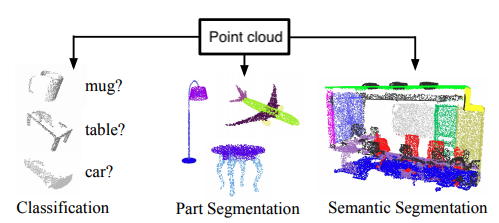# Classifying 3D shapes¶

Techniques for analyzing 3D shapes are becoming increasingly important due to the vast number of sensors such as LiDAR that are capturing 3D data, as well as numerous computer graphics applications. These raw data are typically collected in the form of a point cloud, which corresponds to a set of 3D points $$\{p_i | i = 1, \ldots, n\}$$, where each point $$p_i$$ is a vector of its $$(x, y, z)$$ coordinates plus extra feature channels such as color, intensity etc. Typically, Euclidean distance is used to calculate the distances between any two points.

By finding suitable representations of these point clouds, machine learning can be used to solve a variety of tasks such as those shown in the figure below.This notebook examines how giotto-tda can be used to extract topological features from point cloud data and fed to a simple classifier to distinguish 3D shapes.

If you are looking at a static version of this notebook and would like to run its contents, head over to GitHub and download the source.

## Generate simple shapes¶

To get started, let’s generate a synthetic dataset of 10 noisy circles, spheres, and tori, where the effect of noise is to displace the points that sample the surfaces by a random amount in a random direction:

from data.generate_datasets import make_point_clouds

point_clouds_basic, labels_basic = make_point_clouds(n_samples_per_shape=10, n_points=20, noise=0.5)
point_clouds_basic.shape, labels_basic.shape

((30, 400, 3), (30,))


Here the labels are defined to that a circle is 0, a sphere is 1, and a torus is 2. Each point cloud corresponds to a sampling of the continuous surface – in this case 400 points are sampled per shape. As a sanity check, let’s visualise these points clouds using giotto-tda’s plotting API:

from gtda.plotting import plot_point_cloud

plot_point_cloud(point_clouds_basic)